Next: Longitudinal-field measurements Up: 4.3 SR measurements Previous: 4.3.1 The 3-leg ladder

### Zero-field measurements

Fig.29a shows typical zero-field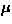SR spectra in the 3-leg ladder system. Below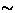55 K, there observed muon spin relaxation, which suggests a magnetic order of the Cu moments. The signal amplitude was large enough to conclude that it comes from the ladder structure, rather than from the CuO impurity phase. The spectra below 45 K converge to 1/3 of the total amplitude; this 1/3-component' is a signature of static order in polycrystalline specimens (see section 3.1). The spectra below 30 K exhibited a dip' between the initial decay and the 1/3-component; the existence of such a dip is another signature of a static order. The solid line for the 50 mK data is a phenomenological fit with the Gaussian Kubo-Toyabe function, which is appropriate to randomly oriented, frozen dense spin systems (see section 3.1).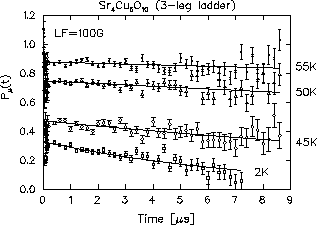The spectra near the transition temperature (Fig.29a) behave as if there is a distribution of ordering temperatures: (1) the relaxation amplitude (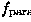ordered volume fraction) decreases as temperature increases and (2) the dip' in the spectra disappears above40 K, although the relaxation is static as shown by the decay to the 1/3-component. The absence of the dip in the static relaxation suggests a distribution of the field-widths (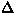), which would result from a distribution in the ordering temperatures. We analyzed theSR spectra with a functional form of: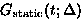(4)
where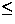is the paramagnetic volume fraction in the sample and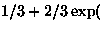is the static Gaussian Kubo-Toyabe function at T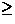30 K or a static Gaussian function (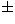)) at T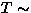40 K. The parameteris the Gaussian field distribution width, which is proportional to the size of the ordered Cu moments. At T40 K,represents an average' of the field-widths at the muon sites. We show the temperature dependence ofandin Fig.29b. The ordering temperature is around 52 K with a distribution of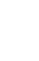5 K, as deduced from the temperature dependence of.Next: Longitudinal-field measurements Up: 4.3 SR measurements Previous: 4.3.1 The 3-leg ladder